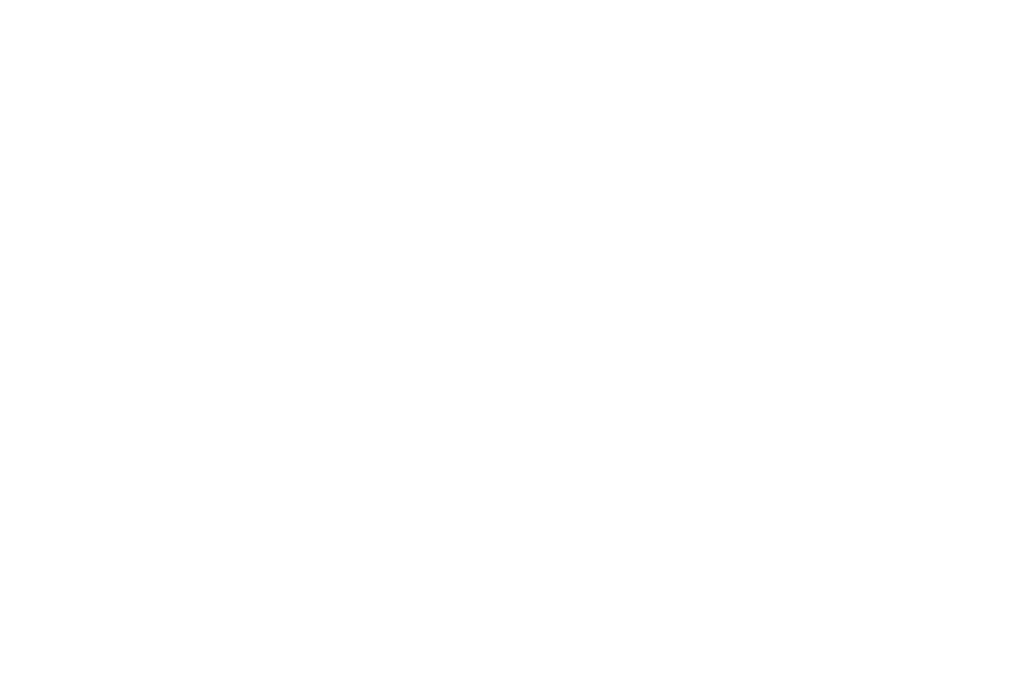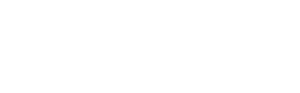# Introduction to Limits of Accuracy | Cambridge IGCSE Mathematics[Please watch the video attached at the end of this blog for a visual explanation of the limits of accuracy]

This lesson is closely linked to the lesson rounding numbers we learned before. In fact, you can think of this as something we do before we round a number up or down.

## What are the limits of accuracy?

To demonstrate all the possible values that a rounded number could have or be, we rely on limits of accuracy, which can adobe referred to as bounds of accuracy.

### Lower bound/ limit

This is the smallest number that could be rounded up to the estimated number.

### Upper bound/ limit

This is the smallest number that could be rounded up to the next estimated number.

Ex:

If we consider 17.5, and we are asked to round it to the nearest whole number, the answer is considered to be 18.

At the same time, if we are asked what gets rounded down to 18, the answer will be anything less than 18.5 will get rounded down to 18. This means even a number such as 18.4999 will get rounded down to 18.

In this example, what can we consider as the two limits of accuracy?

• The smallest value that can be rounded up to 18 will be the lower limit and the highest number that can be rounded down to 18 will be the higher limit.

(In case you need a quick refresher on rounding numbers, you can access our article on it here!)

Ex: 105 cm has been rounded to the nearest centimeter. What could the smallest and largest length of this table be?

In order to find an answer to this, we need to begin by finding out what the value of the table length could have been before it was rounded up or down.

When looking at the possibilities

• it could have been 104.5 because this would have rounded up to 105 cm to the nearest centimeter.

• Or it can be something as big as 105.4999 which we could have rounded down to get 105 cm.

However, it is very difficult to give a specific value for 9999…, as it could be a never-ending value. Therefore, for ease of answering these types of questions, we consider the highest limit to be 105.5cm

This is where it might get a little confusing, so be careful. 105.5 should generally be rounded up to the next number 106, but in limits of accuracy, we consider 105.5 to be the higher limit of accuracy because it is easier to write rather than having many nines in the answer.

Ex 2: Calculate the upper bound of the following:

14*73 / 20

In a calculation like this where each number has been rounded to the nearest centimeter, what are the steps that we must take?

1. Looking at the numerator, if you need the biggest value possible out of this calculation, then the biggest numerator possible for this fraction is what is needed.

(In fractions, the higher the numerator and lower the denominator, the greater the answer will be.

Therefore to get the biggest possible numerator here, we must make sure that 14 and 73 both have the biggest values possible before being rounded up. This means we need to consider the upper limit of accuracy for both 14 and 73.

Going by what we learned earlier, the upper limits of accuracy for 14 and 73 are 14.5 and 73.5 respectively.

2. We must then consider the denominator.

When the denominator of a fraction gets bigger, the decimal value gets smaller. For example, when dividing 1 by 2 (½), the answer is o.5. However, if 1 is divided by 4, the answer is 0.25. We can see how the value gets smaller when the denominator is larger.

Hence, we want the smallest denominator possible to make sure that the entire fraction as a whole is as big as possible. Hence when considering the denominator, we must take the lower limit of accuracy of that value. We will not be considering the denominator to be 20.5, instead, we will take the denominator as 19.5 cm.

3. Once these values are substituted in the calculator, the answer is 54.7 which is the upper bound of this calculation.## Let’s make revision easier!

Read the question carefully. Are they asking for the upper bound of the final calculation? Are they asking about the upper bound of the values? Or are they asking something entirely different? Depending on the question, you will have to change your answer, so pay attention to how the question is worded.

It would be ideal if you can get your hands on past paper questions and attempt them. Some questions can be found here as well, and you can time your answers to see if you can stick to the time limit given.

If you are struggling with IGCSE revision or Mathematics in particular, you can reach out to us at Tutopiya to join revision sessions or find yourself the right tutor for you.

Watch the video below for a visual explanation of the limits of accuracy. Make sure to attempt the quiz once you’re done!